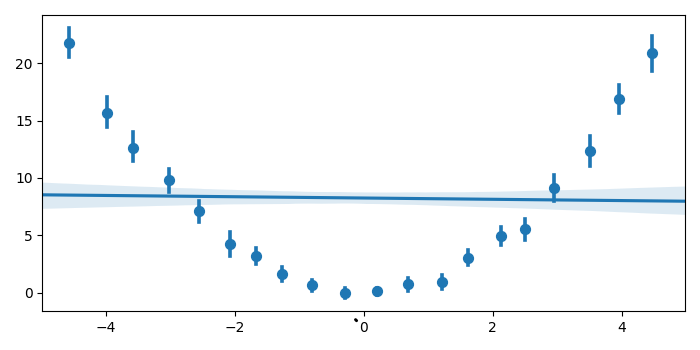# Plotting profile histograms in Python Matplotlib

In a profile histogram, each bin contains the mean of its entries. To plot profile histograms in Python, we can use the regplot method from Seaborn.

## Steps

• Set the figure size and adjust the padding between and around the subplots.

• Create x and y data points using numpy.

• Use seaborn.regplot to plot the data and a linear regress model fit. Use the parameter x_bins to bin the x variable into discrete bins. Use fit_reg=True to plot the regression model relating the x and y variables.

• To display the figure, use Show() method.

## Example

import numpy as np
import seaborn as sns
from matplotlib import pyplot as plt

plt.rcParams["figure.figsize"] = [7.00, 3.50]
plt.rcParams["figure.autolayout"] = True

x = np.random.uniform(-5, 5, 1000)
y = np.random.normal(x**2, np.abs(x) + 1)

sns.regplot(x=x, y=y, x_bins=20, marker='o', fit_reg=True)

plt.show()



## Output

It will produce the following output −Updated on: 19-Oct-2021

364 Views